# Measurement Errors

In this section we discuss validity and reliability in the context of measurement error. There are two types of measurement error: systematic and random . Systematic measurement error is associated with validity; random error is associated with reliability. Let us revisit our example about the bathroom weight scale with an offset of 10 lb. Each time a person uses the scale, he will get a measurement that is 10 lb. more than his actual body weight, in addition to the slight variations among measurements. Therefore, the expected value of the measurements from the scale does not equal the true value because of the systematic deviation of 10 lb. In simple formula:In a general case:where M is the observed /measured score, T is the true score, s is systematic error, and e is random error.

The presence of s (systematic error) makes the measurement invalid. Now let us assume the measurement is valid and the s term is not in the equation. We have the following: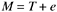The equation still states that any observed score is not equal to the true score because of random disturbance ”the random error e . These disturbances mean that on one measurement, a person's score may be higher than his true score and on another occasion the measurement may be lower than the true score. However, since the disturbances are random, it means that the positive errors are just as likely to occur as the negative errors and these errors are expected to cancel each other. In other words, the average of these errors in the long run, or the expected value of e, is zero: E ( e ) = 0. Furthermore, from statistical theory about random error, we can also assume the following:

• The correlation between the true score and the error term is zero.
• There is no serial correlation between the true score and the error term.
• The correlation between errors on distinct measurements is zero.

From these assumptions, we find that the expected value of the observed scores is equal to the true score:The question now is to assess the impact of e on the reliability of the measurements (observed scores). Intuitively, the smaller the variations of the error term, the more reliable the measurements. This intuition can be observed in Figure 3.4 as well as expressed in statistical terms: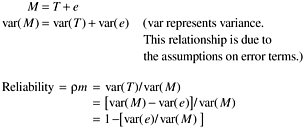Therefore, the reliability of a metric varies between 0 and 1. In general, the larger the error variance relative to the variance of the observed score, the poorer the reliability. If all variance of the observed scores is a result of random errors, then the reliability is zero [1 “ (1/1) = 0].

3.5.1 Assessing Reliability

Thus far we have discussed the concept and meaning of validity and reliability and their interpretation in the context of measurement errors. Validity is associated with systematic error and the only way to eliminate systematic error is through better understanding of the concept we try to measure, and through deductive logic and reasoning to derive better definitions. Reliability is associated with random error. To reduce random error, we need good operational definitions, and based on them, good execution of measurement operations and data collection. In this section, we discuss how to assess the reliability of empirical measurements.

There are several ways to assess the reliability of empirical measurements including the test/retest method, the alternative-form method, the split- halves method, and the internal consistency method (Carmines and Zeller, 1979). Because our purpose is to illustrate how to use our understanding of reliability to interpret software metrics rather than in-depth statistical examination of the subject, we take the easiest method, the retest method. The retest method is simply taking a second measurement of the subjects some time after the first measurement is taken and then computing the correlation between the first and the second measurements. For instance, to evaluate the reliability of a blood pressure machine, we would measure the blood pressures of a group of people and, after everyone has been measured, we would take another set of measurements. The second measurement could be taken one day later at the same time of day, or we could simply take two measurements at one time. Either way, each person will have two scores. For the sake of simplicity, let us confine ourselves to just one measurement, either the systolic or the diastolic score. We then calculate the correlation between the first and second score and the correlation coefficient is the reliability of the blood pressure machine. A schematic representation of the test/retest method for estimating reliability is shown in Figure 3.5.

Figure 3.5. Test/Retest Method for Estimating Reliability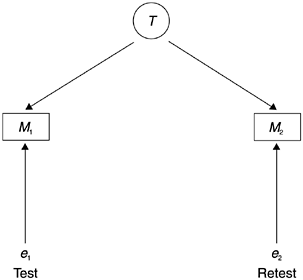The equations for the two tests can be represented as follows :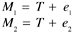From the assumptions about the error terms, as we briefly stated before, it can be shown that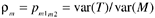in which r m is the reliability measure.

As an example in software metrics, let us assess the reliability of the reported number of defects found at design inspection. Assume that the inspection is formal; that is, an inspection meeting was held and the participants include the design owner, the inspection moderator, and the inspectors. At the meeting, each defect is acknowledged by the whole group and the record keeping is done by the moderator. The test/retest method may involve two record keepers and, at the end of the inspection, each turns in his recorded number of defects. If this method is applied to a series of inspections in a development organization, we will have two reports for each inspection over a sample of inspections. We then calculate the correlation between the two series of reported numbers and we can estimate the reliability of the reported inspection defects.

3.5.2 Correction for Attenuation

One of the important uses of reliability assessment is to adjust or correct correlations for unreliability that result from random errors in measurements. Correlation is perhaps one of the most important methods in software engineering and other disciplines for analyzing relationships between metrics. For us to substantiate or refute a hypothesis, we have to gather data for both the independent and the dependent variables and examine the correlation of the data. Let us revisit our hypothesis testing example at the beginning of this chapter: The more effective the design reviews and the code inspections as scored by the inspection team, the lower the defect rate encountered at the later phase of formal machine testing.

As mentioned, we first need to operationally define the independent variable (inspection effectiveness) and the dependent variable (defect rate during formal machine testing). Then we gather data on a sample of components or projects and calculate the correlation between the independent variable and dependent variable. However, because of random errors in the data, the resultant correlation often is lower than the true correlation. With knowledge about the estimate of the reliability of the variables of interest, we can adjust the observed correlation to get a more accurate picture of the relationship under consideration. In software development, we observed that a key reason for some theoretically sound hypotheses not being supported by actual project data is that the operational definitions of the metrics are poor and there are too many noises in the data.

Given the observed correlation and the reliability estimates of the two variables, the formula for correction for attenuation (Carmines and Zeller, 1979) is as follows: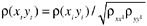where

r ( x t y t ) is the correlation corrected for attenuation, in other words, the estimated true correlation

r ( x i y i ) is the observed correlation, calculated from the observed data

r xx ' is the estimated reliability of the X variable

r yy ' is the estimated reliability of the Y variable

For example, if the observed correlation between two variables was 0.2 and the reliability estimates were 0.5 and 0.7, respectively, for X and Y , then the correlation corrected for attenuation would be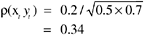This means that the correlation between X and Y would be 0.34 if both were measured perfectly without error.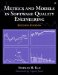Metrics and Models in Software Quality Engineering (2nd Edition)
ISBN: 0201729156
EAN: 2147483647
Year: 2001
Pages: 176